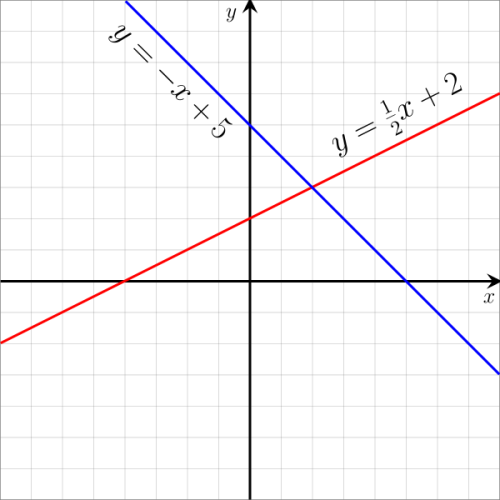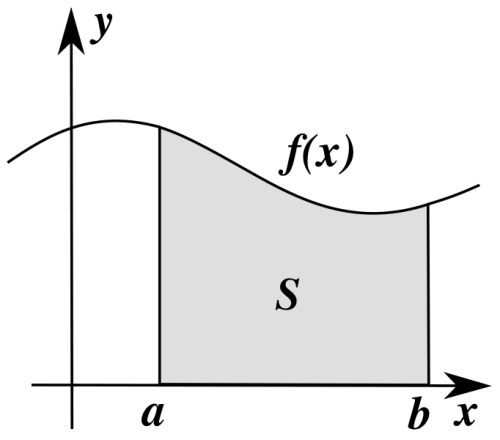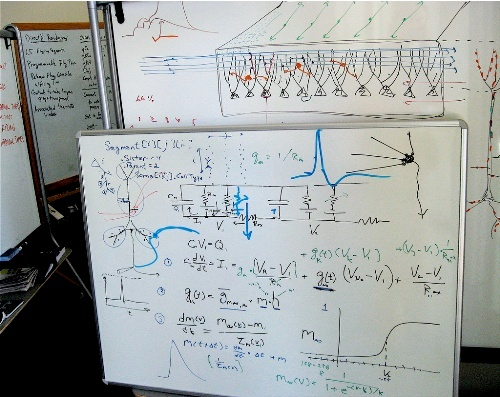# 10 Facts about Linear Functions

Post On: February 1, 2017
By: Agustina

Facts about Linear Functions highlight an interesting topic in mathematics.  This term is defined as related, yet different notions.  It is called as a linear map in the field of algebra and functional analysis. On the other hand, it has a different definition in calculus and other related fields. The people call it as zero polynomial.   In other word, it is used to define the polynomial function of a degree 0 or 1. Why don’t you check the complete facts about linear functions below?

## Facts about Linear Functions 1: a constant function

A linear function is seen on the constant function. It is because this function is called as the zero polynomial or the polynomial of degree zero.Linear function

### Facts about Linear Functions 2: a horizontal line

A horizontal line is used to draw the graph of linear function if it only has an independent variable.

Check Also: 10 Facts about Line Graph

## Facts about Linear Functions 3: a linear map

A linear map is associated with functional analysis and algebra. It is often defined in distinct meaning. People often call it as a linear form or homogenous linear function.

### Facts about Linear Functions 4: in linear algebra

Linear function is referred to a special type of affine map when you define this term in linear algebra context.## Facts about Linear Functions 5: the linear function and linear map

The linear maps should be the values of scalar field if it earns the term linear function according to several writers. People also name this situation as a linear functional.

### Facts about Linear Functions 6:  the straight line

The straight line is found in the graphs of a linear function where it does not have any powers or exponents. However, it has one or two variables.Linear Functions Work

## Facts about Linear Functions 7: the form of linear function

Have you realized the form of linear function?  It is written in f(x) = a + bx.  It has a straight line on the graph. Moreover, it owns a dependent variable and one independent variable. The y refers to the dependent variable, while x is the independent one.

### Facts about Linear Functions 8: the importance of linear function

Linear function is very important in calculus for it serves as the basic function in the field.

## Facts about Linear Functions 9: the graph

When you want to draw linear function in a graph, it only has two points reflected in (a, f(a)), (b, f(b)).